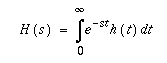Mathematical Equations
[Top] [Up] [Next] [Previous]

# 12 - Mathematical Equations

Currently, the best way of including equations in HTML documents is to first write the document in LaTeX and then use the latex2html filter to create the corresponding HTML document, together with the equations as a number of bitmap files*1. The previous draft of the HTML+ specification described a way of embedding LaTeX equations in HTML+ documents. Unfortunately, it now seems too cumbersome to form a practical solution, and has been dropped.

The following is a preliminary proposal for representing equations directly as HTML+ using an SGML-based notation, inspired by the approach taken by LaTeX. It is intended to cover the majority of users needs, rather than aiming for complete coverage. This makes it practical to use a simplified notation compared with richer notations, e.g. the ISO 12083 Maths DTD. An experimental browser supporting the MATH element is being developed at CERN.

Consider the equation:This can be represented as:

```[itex]
H(s) = ∫<sub>0</sub><sup>∞</sup> e<sup>-st</sup> h(t) dt
[/itex]
```
The mathematical symbols are given with their standard ISO entity names. SUB and SUP are used to specify subscripts and superscripts. For integral signs and related operators, the subscript/superscript text is centered over the symbol, otherwise it appears to the right as shown in the preceding example. The BOX and OVER elements allow you to define more complex equations, as in:which is represented by:

```[itex]
C <box>dV<sub>out</sub><over>dt</box> = I<sub>b</sub>
&tanh;(<box>κ(V<sub>in</sub>-V<sub>out</sub>)<over>2</box>)
[/itex]
```
The BOX element can be used to generally group items and can be thought of as non-printing parentheses. The OVER element is optional and divides the box into numerator and denominator. The ARRAY element is used to specify arrays for expressions like:The ARRAY element has a single attribute ALIGN which specifies the number of columns and the alignment of items within the columns. For each column there is a single letter that specifies how items in that column should be positioned: c for centered, l for flush left or r for flush right. Each item in the array must follow an <ITEM> element.

The preceding example is represented by:

```[itex]
(<array align="c"> <item>
&ldet;<array align="cc">
<item>x<sub>11</sub>
<item>x<sub>12</sub>
<item>x<sub>21</sub>
<item>x<sub>22</sub>
</array><rd>&rdet;
<item> y <item> z
</array>)
[/itex]
```
The browser is responsible for working out the vertical and horizontal spacing required for the array. Parentheses*2 are stretched to match the size of the array. Arrays can be used only in the context of the MATH element. The TABLE element should be used for other contexts.

Spaces are significant within the MATH element, and used for disambiguation, as can be seen in the following two examples:Authors can adjust the default horizontal spacing with the ISO entities: &thinsp; for thin space (1/6 em) and &hairsp; for hair space. Horizontal, diagonal and vertical ellipsis are possible with &hellip; &dellip; and &vellip; respectively. Common functions like sin, log and tanh should be rendered in a non-italic font. These functions are defined by their entity namesakes. Additional elements are needed to represent roots and for over and under lining.

An open question is how to render maths on dumb terminals. One approach is to translate into an existing convention such as Mathematica. Another is to write equations as they would be spoken aloud. For GUI displays, browsers need to be able to show characters in at least two point sizes as well as being able to stretch parentheses and integral signs etc. to various sizes. The processing time needed to size and position symbols suggests that caching may be useful to speed up subsequent scrolling and refresh operations.

Comments from mathematicians are welcomed. Widespread support for formulae is likely to be delayed until most platforms support the relevant symbols fonts (or Unicode).

HTML+ Discussion Document - November 8, 1993